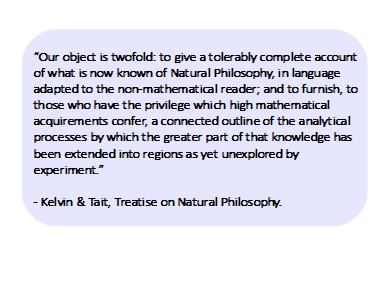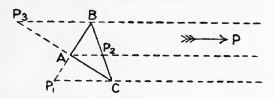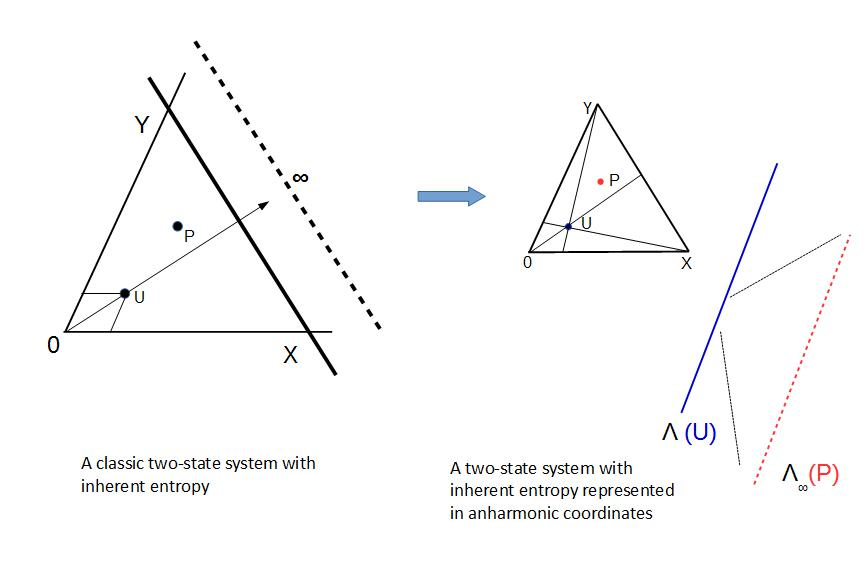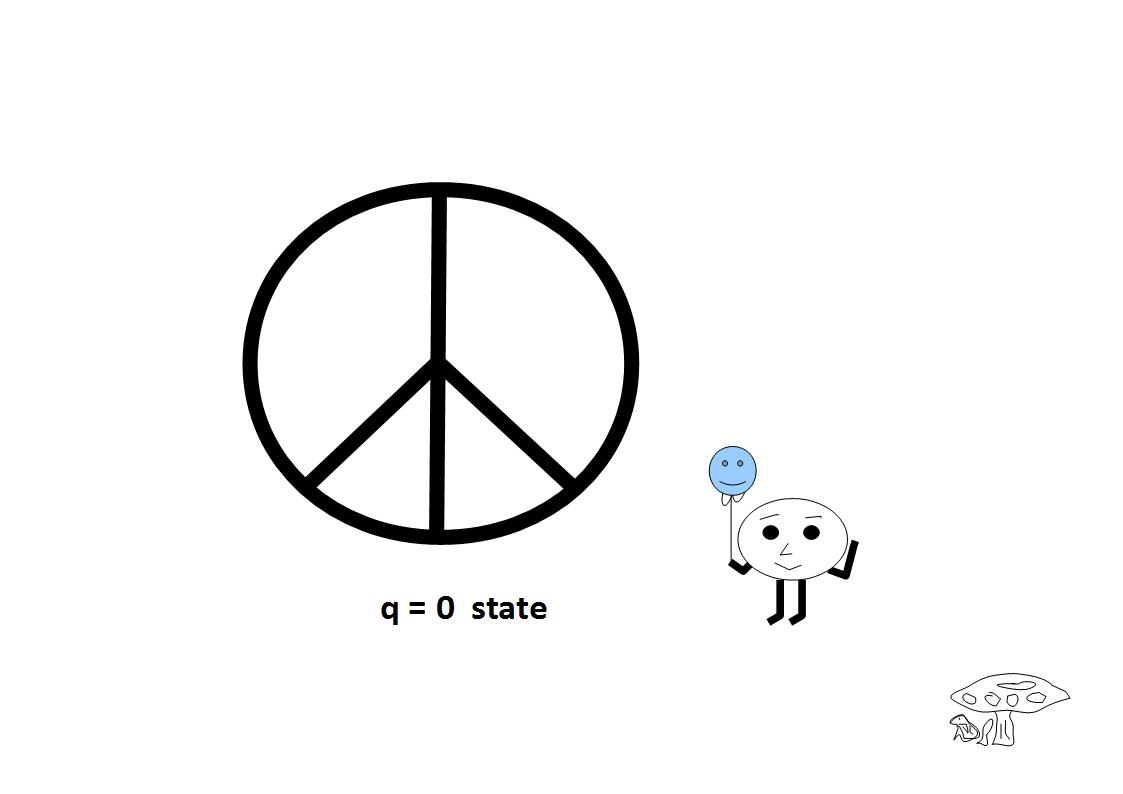# ijspace.org

The Rationale behind Irrational

9th
August 2015

The idea of anharmonic coordinates was developed by Hamilton based on barycentric calculus by Möbius. The barycentric calculus provides the description of a system with respect to its center of gravity as origin. If we take a triangle and placed weights on its vertices, then we can find a point P4 within the triangle which will behave as the center of gravity. We can increase the weights so that the ratio between the weights remains constant then the position of point P4 remains unchanged. Therefore rather than being restricted to just three points in Cartesian reference frame we have many more points describing the system.Further in the case of Cartesian coordinates the origin may be independent of the vertices or the triangle, whereas in barycentric coordinate system we can choose the point P4 within the triangle as reference and describe the system with respect to it.

In anharmonic coordinates we describe the measurements with unit-point U as a reference.
Next we have the projective coordinates which are the coordinates from a projective transformation. The projective coordinates preserve the cross-ratio of four non-collinear points (P1, P2, P3, P4). From this definition the Mö
bius  net can be generated. For details please refer to Fauvel  et. al., the essay titled Möbius's Geometrical Mechanics. Here we should also note that we used similar concept of two-state system, (e-h, e+h), to develop knot-polynomials. However a much better understanding of z-axis is obtained with anharmonic coordinates. Please remember the most important construct is, how we draw the line XY to form the measurement triangle ΔOXY and then define the unit-point U.We are developing similar description for a two-state system in the form of a geometrical net constructed from the measurements made by an observer of infinitesimal capacity. We have provided the definition of unit point and unit line. The correlation to the "constructability" and the measurement-net in anharmonic coordinates is also discussed. Next is the description of an arbitrary point P denoted as (x, y, z). Consider the case that point P is a rational point i.e. it falls on the geometrical net. The unit-point is denoted by U, then define þ = UP given by,

þ = (xlα + ymβ + znγ)/ (xl + ym +zn);

where x, y, and z are whole numbers.
Similarly if the unit point is irrational, the þ is given by,

þ = (pα + qβ + rγ)/ (p + q + r);

where p, q, r are constructed similar to l, m, and n, with respect to P instead of U.

Finally we perform another very important construct that of a polar [x, y, z] to the arbitrary point P (x, y, z) as pole, denoted by
Λ
.The importance of Λ can not be underestimated as it represents the macroscopic observer ObsM, i.e. all-knowing us and our (ct, x, y, z) metric. Λ
is defined by the equation,

lx + my + nz = 0.

A very nice geometrical representation of
Λ
, is provided by Hime and it is reproduced below (Figure-1).Figure-1: The geometrical representation of Λ.

Please note that for the above construction for
Λ to be true, the point P has to be an irrational point which is essentially a rational point on the geometric net under the infinite limit.Further for the observer with the iconic tail and horns i.e. Obsi, the unit lines for U and P represented by Λ and Λ, collapse to the unit point U which implies a single measurement or a zero-entropy measurement. Our basic setup of a geometric net of measurements is shown in Figure-2. Please note that all the structures represent the information content. Therefore a straight line in information space is not necessarily a straight line in Euclidean space.Figure-2: The basic geometric structure in measurement space based on Anharmonic Coordinates. The macroscopic observer is represented by Λ.

For the macroscopic observer ObsM the unit point U represents the measurement of an information space with infinite content. Therefore ObsM can never measure the unit point U. Furthermore the unit-point U represents the only structure with infinite life-time per measurements of ObsM or the true PE1 measurement. Any other structure(s) measured by ObsM will be represented by the point P, rational or irrational values
, all of which will be structures with finite life-time or PE<1 (rational values of P) and PE<<1 (irrational values of P) events*. We can also say as we did before, that anything we can measure will always be temporary (protons, electrons, sub-atomic particles etc.) and whatever is permanent we can not measure.

What we have done essentially is to link two different types of representations. One in which the observer's capacity is not an issue in developing the physical description, such as Euclidean space or Cartesian coordinates which are represented by the point P and
Λ. Second in which the observer's limitations are accounted for. Examples of these representations are Barycenteric Coordinates and Anharmonic Coordinates which resulted in the structures such as ΔOXY, unit-point U, and the unit-line Λ. Consequently it has allowed us some understanding of rational and irrational numbers and how they relate to an observer with finite capacity, which is extremely important. Another important concept is the definition of the "constructibility". The constructibility by compass and straight edge as defined in field theory does not worry about the space and hence it is not suited for the description of a two-state system. We are able to account for space in constructibility by using anharmonic coordinates. We will look into this aspect in greater detail in future blogs.

On a lighter note let us assume we can build a mechanism (a computer if we may) which is supposed to measure the entire universe.The universe to us implies q = 3 state which represents electron-photon interaction as the basic mechanism for measurement of the universe. It is built in (ct, x, y, z) metric and it obviously can not work at the light speed efficiency. So if it can not measure the universe in one measurement do we really think that it will be able to measure all the information contained within the universe eventually? Good Luck! Please have your plum pudding before it is too late.

There is extensive work done by Hamilton and later by Hime on the geometrical properties related to conic sections (the locus of point P) and cross-ratios in anharmonic coordinates. It is a pity that the work by others in this area are not known to us.
We will discuss the Pauli's spin-matrices next.

*Note - PE1 measurement: Probability Equal to 1 measurement;  PE<1 measurement: Probability Equal to less than 1 measurement; etc.

To be continued....Previous Blogs: